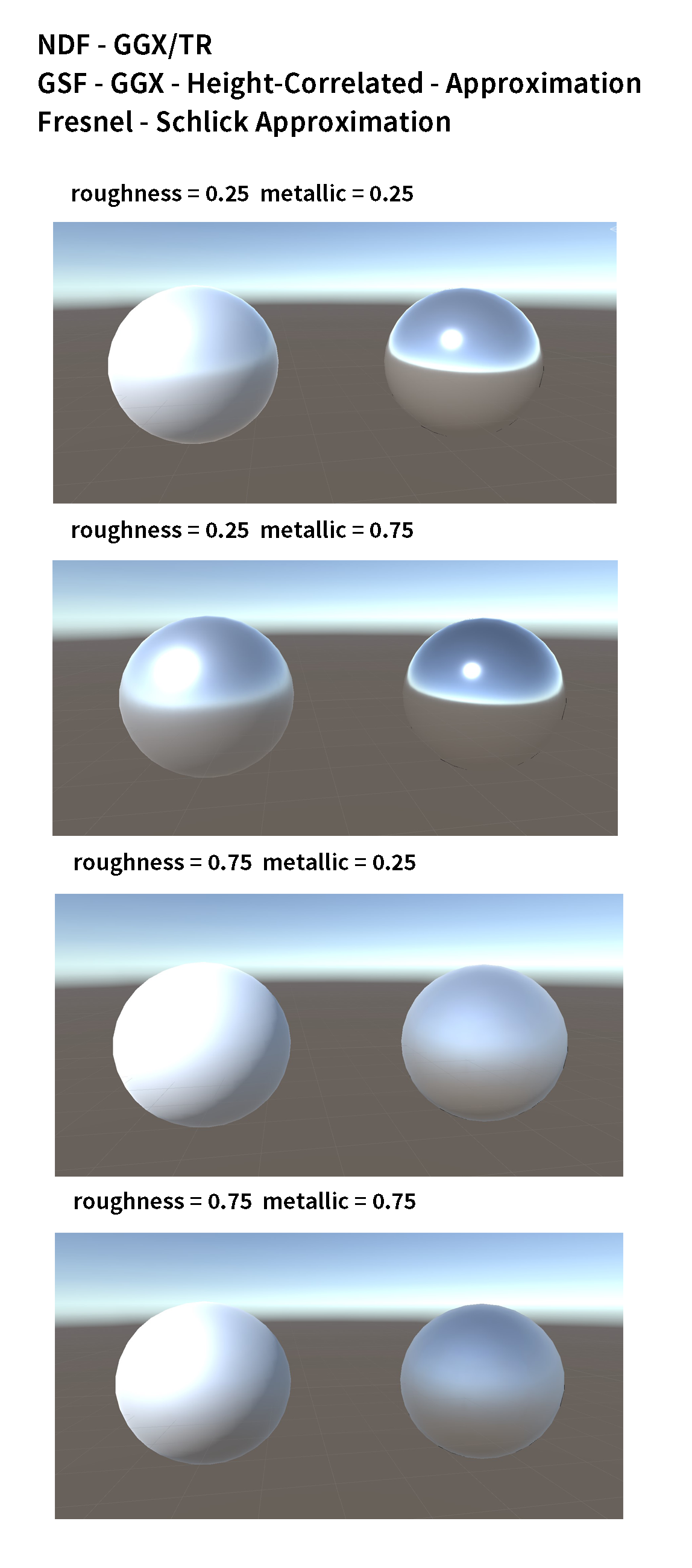## 环境光照的漫反射项

$\frac{\rho_{\mathrm{ss}}}{\pi} k_{\mathrm{diffuse}} \int_{\Omega} L(l) (l \cdot n) \mathrm{d}l$

## 环境光照的镜面反射项

$\int_{\Omega} f(l, r) L(l) (l \cdot n) \mathrm{d} l \approx \int_{\Omega} L(l) \mathrm{d} l \cdot \int_{\Omega} f(l, v) (l \cdot n) \mathrm{d} l$

$\int_{\Omega} f(l, v) (l \cdot n) \mathrm{d} l$

$= \int_{\Omega} D(m) V(l, v) F(v, m) (l \cdot n) \mathrm{d} l$

$= \int_{\Omega} D(m) V(l, v) (l \cdot n) (F_0 + (1 - F_0) (1 - (n \cdot v))^5) \mathrm{d} l$

$= F_0 \int_{\Omega} D(m) V(l, v) (l \cdot n) (1 - (1 - (n \cdot v))^5) \mathrm{d} l + \int_{\Omega} D(m) V(l, v) (l \cdot n) (1 - (n \cdot v))^5 \mathrm{d} l$

$= F_0 \times A + B$

## 在 Unity 中获取环境光照信息

Unity 内置了一套获取环境光照信息的工具，Stevens 的教程中给出了使用这套工具的代码：

## 整合结果与对比

NDF 使用 GGX/TR 分布、GSF 使用 GGX Smith 高度相关遮蔽阴影函数的近似，菲涅尔项使用 Schlick 近似，漫反射项使用 Disney 漫反射，环境光照使用 Unity 提供的，结果如下。其中左侧为 Unity 自己的 PBR（Unity 提供的参数的光泽度而不是粗糙度），右侧是自己实现的 PBR，可以看到区别还是相当的大的。可能原因除了粗糙度的转换关系不同以外，我在调节粗糙度使自己的 PBR 结果看起来和 Unity 的差不多时，发现自己很难达到像它那样那么亮的反光。------ 本文结束，感谢您的阅读 ------